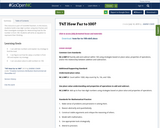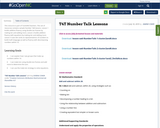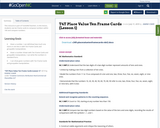Updating search results...

# 3 Results

View
Selected filters:
• frameConditional Remix & Share Permitted
CC BY-NC-SA
Rating
0.0 stars

This resource is part of Tools4NCTeachers.

In this lesson, students build a two digit number using tens frames and then investigate strategies for determining how far the number is from 100. Students will write an equation to represent their thinking.

Subject:
Mathematics
Material Type:
Lesson
Lesson Plan
11/06/2019Conditional Remix & Share Permitted
CC BY-NC-SA
Rating
0.0 stars

This resource is part of Tools4NCTeachers.

This set of resources contains sample Number Talk lessons. Lesson 1 builds addition fluency using double ten-frames by making ten and adding more. Lesson 2 builds addition fluency with equations by making ten and adding more. Lesson 3 uses dot cards as representations of numbers to build math language as well as fluency with decomposing numbers within 10.

Subject:
Mathematics
Material Type:
Activity/Lab
Lesson
Lesson Plan
11/06/2019Conditional Remix & Share Permitted
CC BY-NC-SA
Rating
0.0 stars

This resource is part of Tools4NCTeachers.

In this lesson, students use ten frame cards to compose numbers within 100 and compare numbers.

Subject:
Mathematics
Material Type:
Lesson
Lesson Plan